This notebook is part of the kikuchipy documentation https://kikuchipy.org. Links to the documentation won't work from the notebook.

# Pattern matching¶

Crystal orientations can be determined from experimental EBSD patterns by matching them to simulated patterns of known phases and orientations, see e.g. Chen et al. (2015), Nolze et al. (2016), Foden et al. (2019).

Here, we will demonstrate dictionary indexing (DI) using a small Ni EBSD data set and a dynamically simulated Ni master pattern from EMsoft, both of low resolution and found in the kikuchipy.data module. The pattern dictionary is generated from a uniform grid of orientations with a fixed projection center (PC). The true orientation is likely to fall in between grid points, which means there is always a lower angular accuracy associated with DI. We can improve upon each solution by letting the orientation deviate from the grid points. We do this by maximizing the similarity between experimental and simulated patterns using numerical optimization algorithms from the SciPy library. This is here called orientation refinement. We could instead keep the orientations fixed and let the PC parameters deviate from their fixed values used in the dictionary, here called projection center refinement. Finally, we can also refine both at the same time, here called orientation and projection center refinement. The need for orientation or orientation and PC refinement is discussed by e.g. Singh et al. (2017), Winkelmann et al. (2020), and Pang et al. (2020).

The term pattern matching is here used for the combined approach of DI followed by refinement.

Before we can generate a dictionary of simulated patterns, we need a master pattern containing all possible scattering vectors for a candidate phase. This can be simulated using EMsoft (Callahan and De Graef (2013) and Jackson et al. (2014)) and then read into kikuchipy.

First, we import libraries and load the small experimental Nickel test data

In :
# Exchange inline for notebook or qt5 (from pyqt) for interactive plotting
%matplotlib inline

import tempfile
import matplotlib.pyplot as plt
import hyperspy.api as hs
import numpy as np
from orix import sampling, plot, io
from orix.vector import Vector3d
import kikuchipy as kp

plt.rcParams.update({"figure.facecolor": "w", "font.size": 15})

s

Out:
<EBSD, title: patterns Scan 1, dimensions: (75, 55|60, 60)>

To obtain a good match, we must increase the signal-to-noise ratio. In this pattern matching analysis, the Kikuchi bands are considered the signal, and the angle-dependent backscatter intensity, along with unwanted detector effects, are considered to be noise. See the pattern processing guide for further details.

In :
s.remove_static_background()
s.remove_dynamic_background()

Removing the static background:
[########################################] | 100% Completed |  0.2s
Removing the dynamic background:
[########################################] | 100% Completed |  1.0s

Note DI is computationally intensive and takes in general a long time to run due to all the pattern comparisons being done. To maintain an acceptable memory usage and be done within reasonable time, it is recommended to write processed patterns to an HDF5 file for quick reading during DI.
In :
#s.save("pattern_static_dynamic.h5")


## Dictionary indexing¶

Next, we load a dynamically simulated Nickel master pattern generated with EMsoft, in the northern hemisphere projection of the square Lambert projection for an accelerating voltage of 20 keV

In :
energy = 20
mp = kp.data.nickel_ebsd_master_pattern_small(projection="lambert", energy=energy)
mp

Out:
<EBSDMasterPattern, title: ni_mc_mp_20kv_uint8_gzip_opts9, dimensions: (|401, 401)>
In :
mp.plot()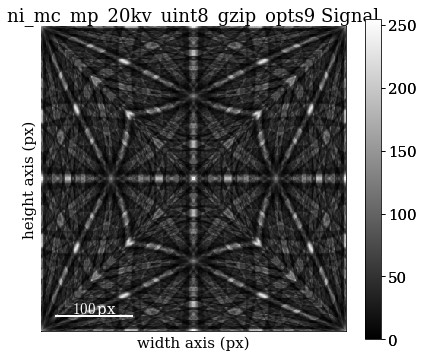The Nickel phase information, specifically the crystal symmetry, asymmetric atom positions, and crystal lattice, is conveniently stored in an orix.crystal_map.Phase

In :
ni = mp.phase
ni

Out:
<name: ni. space group: Fm-3m. point group: m-3m. proper point group: 432. color: tab:blue>
In :
ni.structure  # Element, x, y, z, site occupation

Out:
[28   0.000000 0.000000 0.000000 1.0000]
In :
ni.structure.lattice  # nm and degrees

Out:
Lattice(a=0.35236, b=0.35236, c=0.35236, alpha=90, beta=90, gamma=90)

### Sample orientation space¶

If we don't know anything about the possible crystal (unit cell) orientations in our sample, the safest thing to do is to generate a dictionary of orientations uniformly distributed in a candidate phase's orientation space. To achieve this, we sample the Rodrigues Fundamental Zone of the proper point group 432 using cubochoric sampling Singh and De Graef (2016) available in orix.sampling.get_sample_fundamental(). We can choose the average disorientation between orientations, the "resolution", of this sampling. Here, we will use a rather low resolution of 3$^{\circ}$.

Note Cubochoric sampling became available in orix v0.7.
In :
rotations = sampling.get_sample_fundamental(
method="cubochoric", resolution=3, point_group=ni.point_group
)
rotations

Out:
Rotation (30443,)
[[ 0.8562 -0.3474 -0.3474 -0.1595]
[ 0.8562 -0.3511 -0.3511 -0.1425]
[ 0.8562 -0.3544 -0.3544 -0.1252]
...
[ 0.8562  0.3544  0.3544  0.1252]
[ 0.8562  0.3511  0.3511  0.1425]
[ 0.8562  0.3474  0.3474  0.1595]]

This sampling resulted in 30 443 crystal orientations. See the orix user guide on orientation sampling for further details and options for orientation sampling.

Note An average disorientation of 3$^{\circ}$ results in a course sampling of orientation space; a lower average disorientation should be used for experimental work.

### Define the detector-sample-geometry¶

Now that we have our master pattern and crystal orientations, we need to describe the EBSD detector's position with respect to the sample (interaction volume). This ensures that projecting parts of the master pattern onto our detector yields dynamically simulated patterns resembling our experimental ones. See the reference frames user guide and the EBSDDetector class for further details.

In :
signal_shape = s.axes_manager.signal_shape[::-1]
detector = kp.detectors.EBSDDetector(
shape=signal_shape,
pc=[0.421, 0.7794, 0.5049],
sample_tilt=70,
convention="tsl",
)
detector

Out:
EBSDDetector (60, 60), px_size 1 um, binning 1, tilt 0, azimuthal 0, pc (0.421, 0.221, 0.505)

Let's double check the projection/pattern center (PC) position on the detector using plot()

In :
detector.plot(coordinates="gnomonic", pattern=s.inav[0, 0].data)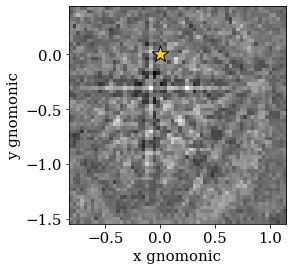### Generate dictionary¶

Now we're ready to generate our dictionary of simulated patterns by projecting parts of the master pattern onto our detector for all sampled orientations, using the get_patterns() method. The method assumes the crystal orientations are represented with respect to the EDAX TSL sample reference frame RD-TD-ND.

Note It is in general adviced to not compute the dictionary immediately, but let the dictionary indexing method handle this, by passing compute=False. This will return a LazyEBSD signal, with the dictionary patterns as a [dask array](https://docs.dask.org/en/latest/array.html).
In :
sim = mp.get_patterns(
rotations=rotations,
detector=detector,
energy=energy,
dtype_out=np.float32,
compute=True
)
sim

Creating a dictionary of (30443,) simulated patterns:
[########################################] | 100% Completed |  3.0s

Out:
<EBSD, title: , dimensions: (30443|60, 60)>

Let's inspect the three first of the 30 443 simulated patterns

In :
#sim.plot()  # Plot the patterns with a navigator for easy inspection
fig, ax = plt.subplots(ncols=3, figsize=(18, 6))
for i in range(3):
ax[i].imshow(sim.inav[i].data, cmap="gray")
ax[i].set_title(
f"($\phi_1, \Phi, \phi_2)$ = {np.array_str(euler, precision=1)}"
)
ax[i].axis("off")
fig.tight_layout()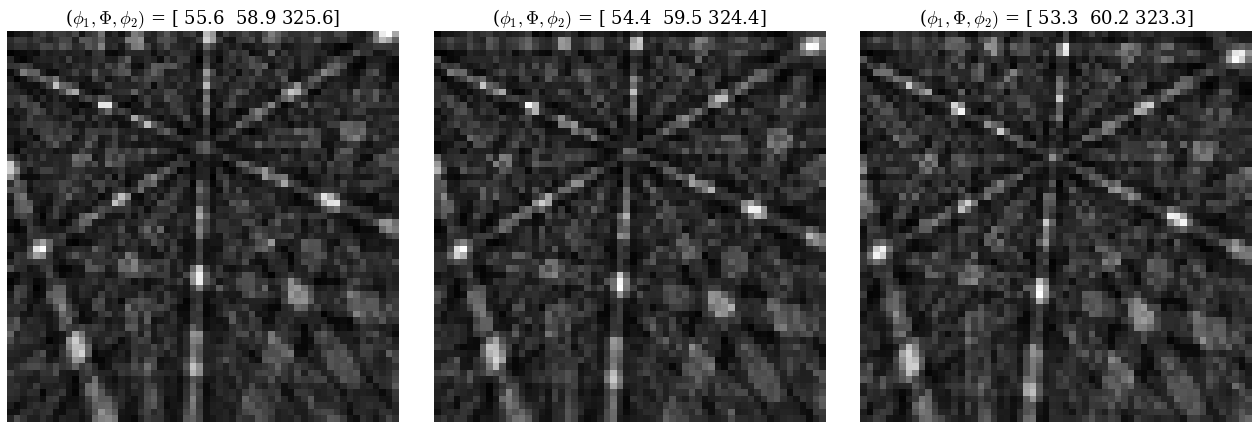### Perform indexing¶

The Kikuchi pattern signal is usually weak towards the corners of the detector, so we can pass a signal mask to only match the pixels where the mask values are False, i.e. mask out the values that are True. This convention is in line with how NumPy, Dask, scikit-image etc. defines a mask. We can pass whatever mask we want, as long as it is a boolean array of the detector shape.

In :
signal_mask = ~kp.filters.Window("circular", signal_shape).astype(bool)

p = s.inav[0, 0].data
fig, ax = plt.subplots(ncols=2, figsize=(10, 5))
ax.set_title("Not used in matching")
ax.set_title("Used in matching");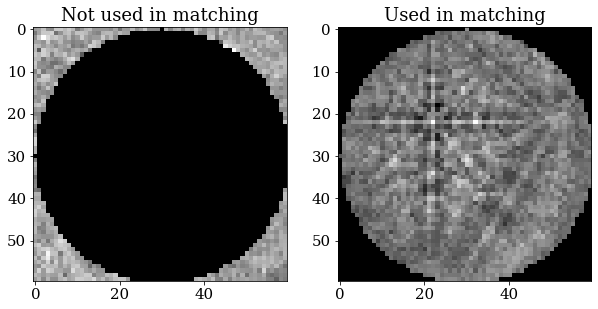Finally, let's use the dictionary_indexing() method to match the simulated patterns to our experimental patterns, using the zero-mean normalized cross correlation (NCC) coefficient $r$ Gonzalez & Woods (2017), which is the default similarity metric. Let's keep the 20 best matching orientations. A number of 4125 * 30443 comparisons is quite small, which we can do in memory all at once. However, in cases where the number of comparisons are too big for our memory to handle, we should iterate over the dictionary of simulated patterns by passing the number of patterns per iteration. To demonstrate this, we do at least 10 iterations here. The results are returned as a orix.crystal_map.CrystalMap.

In :
xmap = s.dictionary_indexing(
sim,
metric="ncc",
keep_n=20,
)
xmap

Dictionary indexing information:
Phase name: ni
Matching 4125 experimental pattern(s) to 30443 dictionary pattern(s)
NormalizedCrossCorrelationMetric: float32, greater is better, rechunk: False, signal mask: True

100%|████████████████████████████████████████████████████████████████████| 11/11 [00:08<00:00,  1.37it/s]

	Indexing speed: 514 patterns/s, 15653977 comparisons/s

Out:
Phase   Orientations  Name  Space group  Point group  Proper point group     Color
0  4125 (100.0%)    ni        Fm-3m         m-3m                 432  tab:blue
Properties: scores, simulation_indices
Scan unit: px
Note *Added in version: 0.5.* Dictionary indexing of real world data sets takes a long time because the matching is computationally intensive. The [dictionary_indexing()](../reference.rst#kikuchipy.signals.EBSD.dictionary_indexing) method accepts parameters *n_per_iteration*, *rechunk* and *dtype* that lets us control this behaviour to a certain extent, so be sure to take a look at the method's docstring.

The normalized dot product can be used instead of the NCC by passing metric="ndp". A custom metric can be used instead, by creating a class which inherits from the abstract class SimilarityMetric.

The results can be exported to an HDF5 file re-readable by orix, or an .ang file readable by MTEX and some commercial packages

In :
temp_dir = tempfile.mkdtemp() + "/"
io.save(temp_dir + "ni.h5", xmap)
io.save(temp_dir + "ni.ang", xmap)


### Analyze indexing results¶

With the orix library we can plot inverse pole figures (IPFs), color orientations to produce orientation maps (also called IPF maps), and more. If we want to inspect the results as grains, orientation density functions, or something else, we have to use other software, like MTEX.

Let's generate an IPF color key and plot it

In :
ckey = plot.IPFColorKeyTSL(ni.point_group)
print(ckey)
ckey.plot()

IPFColorKeyTSL, symmetry: m-3m, direction: [0 0 1]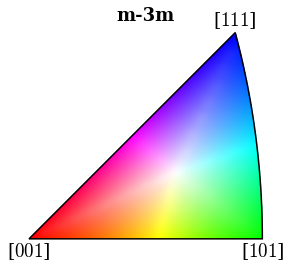With this color key we can color orientations according to which crystal directions the sample direction $Z$ points in in every map pixel

In :
xmap.scan_unit = "um"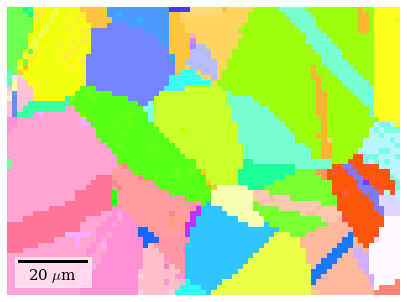With a few more lines, we can plot maps for all three sample directions

In :
ori = xmap.orientations
directions = Vector3d(((1, 0, 0), (0, 1, 0), (0, 0, 1)))

fig, axes = plt.subplots(figsize=(15, 5), ncols=3)
for ax, v, title in zip(axes, directions, ("X", "Y", "Z")):
ckey.direction = v
rgb = ckey.orientation2color(ori).reshape(xmap.shape + (3,))
ax.imshow(rgb)
ax.axis("off")
ax.set_title(f"IPF {title}")
fig.tight_layout()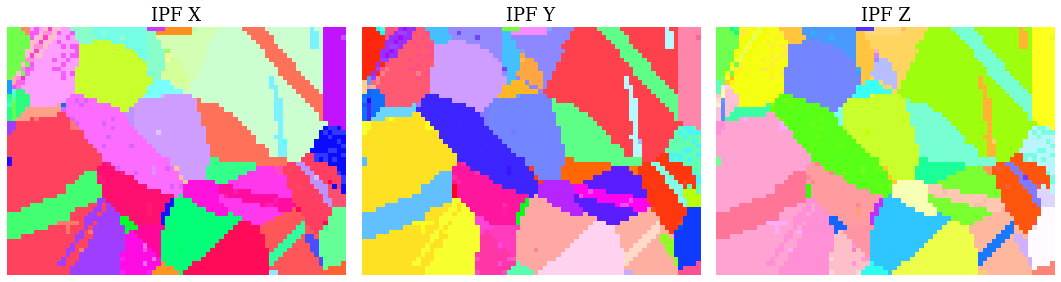The sample is recrystallized Ni, so we expect a continuous color within grains, except for twinning grains. The orientation maps are mostly in line with our expectation. Some grains have a scatter of similar colors, which is most likely due to the discrete nature of our dictionary. To improve upon this result we can reduce the orientation sampling characteristic distance. This will increase our dictionary size and thus our indexing time. An alternative is to perform orientation refinement, which we will do below.

We can get further confirmation of our initial analysis by inspecting some indexing quality maps, like the best matching scores and so-called orientation similarity (OS) map, which compares the best matching orientations for each pattern to it's nearest neighbours. Let's get the NCC map in the correct shape from the CrystalMap’s scores property and the OS map with orientation_similarity_map() using the full list of best matches per point

In :
ncc_map = xmap.scores[:, 0].reshape(*xmap.shape)
os_map = kp.indexing.orientation_similarity_map(xmap)


And plot the maps

In :
fig, ax = plt.subplots(ncols=2, figsize=(10, 3))
im0 = ax.imshow(ncc_map)
im1 = ax.imshow(os_map)
fig.colorbar(im0, ax=ax, label="NCC")
fig.colorbar(im1, ax=ax, label="Orientation similarity")
ax.axis("off")
ax.axis("off")
fig.tight_layout()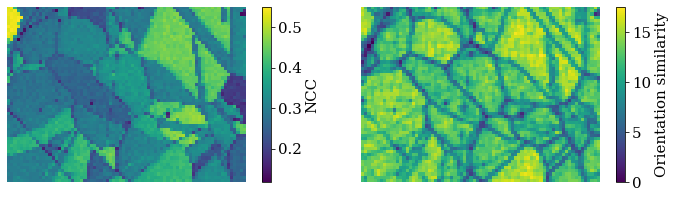From the NCC map we see that some grains match better than others. Due to the discrete nature of our dictionary, it is safe to assume that the best matching grains have patterns more similar to those in the dictionary than the others, that is, the different coefficients does not reflect anything physical in the microstructure. The OS map doesn't tell us much more than the NCC map in this case.

We can use the crystal map property simulation_indices to get the best matching simulated patterns from the dictionary of simulated patterns

In :
best_patterns = sim.data[xmap.simulation_indices[:, 0]].reshape(s.data.shape)
s_best = kp.signals.EBSD(best_patterns)
s_best

Out:
<EBSD, title: , dimensions: (75, 55|60, 60)>

The simplest way to visually compare the experimental and best matching simulated patterns are to plot them in the same navigator. Let's create an RGB navigator signal from the IPF $Z$ orientation map with the convenience function get_rgb_navigator(). When using an interactive backend like Qt5Agg, we can then move the red square around to look at the patterns in each point.

In :
rgb_navigator = kp.draw.get_rgb_navigator(rgb)
hs.plot.plot_signals([s, s_best], navigator=rgb_navigator)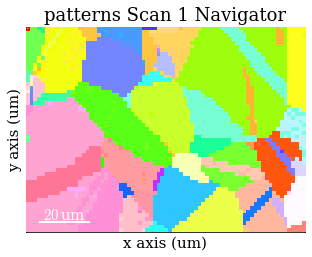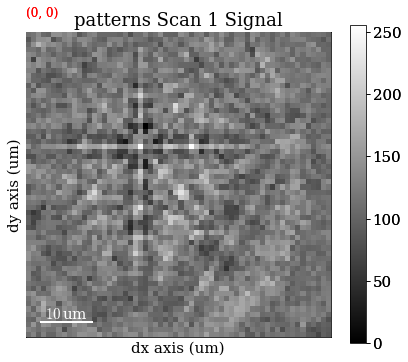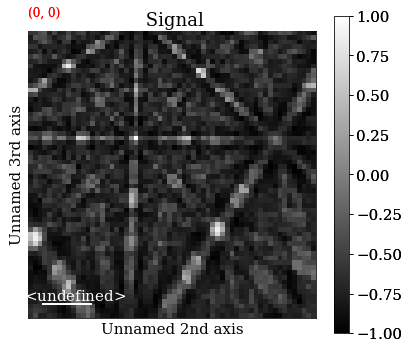Let's also plot the best matches for patterns from two grains

In :
grain1 = (0, 0)
grain2 = (30, 10)
fig, ax = plt.subplots(ncols=2, nrows=2, figsize=(10, 10))
ax[0, 0].imshow(s.inav[grain1].data, cmap="gray")
ax[0, 0].axis("off")
ax[0, 1].imshow(s_best.inav[grain1].data, cmap="gray")
ax[0, 1].axis("off")
ax[1, 0].imshow(s.inav[grain2].data, cmap="gray")
ax[1, 0].axis("off")
ax[1, 1].imshow(s_best.inav[grain2].data, cmap="gray")
ax[1, 1].axis("off")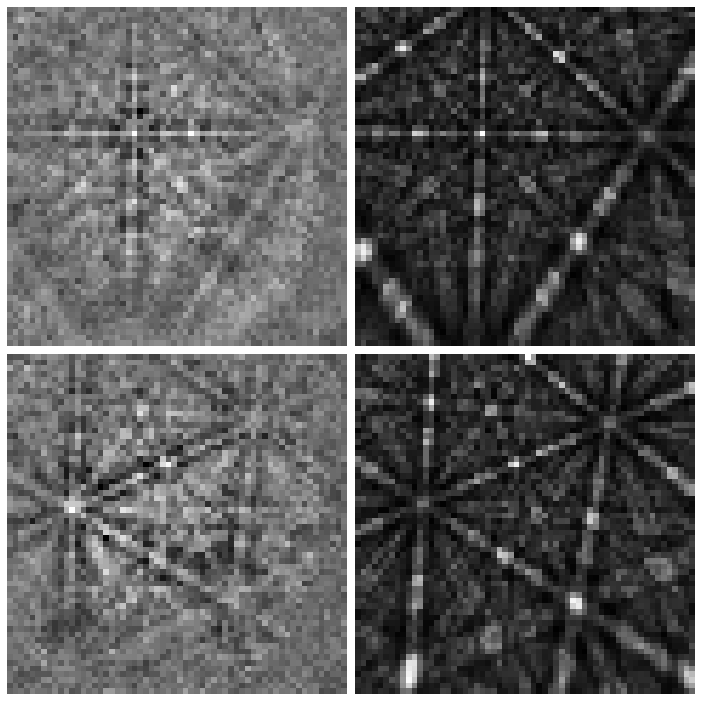## Refinement¶

Let's look at the effect of three refinement routes, all implemented as EBSD methods:

1. Refine orientations while keeping the PCs fixed: refine_orientation()
2. Refine PCs while keeping the orientations fixed: refine_projection_center()
3. Refine orientations and PCs at the same time: refine_orientation_projection_center()

For each run we will compare the maps and histograms of NCC scores before and after refinement, and also the PC parameters if appropriate.

As stated at the top, we use the numerical optimization routines from the SciPy library. For every orientation and/or PC, we want to iteratively increase the similarity (NCC score) between our experimental pattern and a new simulated pattern projected onto our EBSD detector for every iteration until the score increase from one iteration to the next is below a certain threshold, by default 0.0001 in most cases. The orientation and/or PC is updated slightly for every iteration. We have access to both local and global optimization algorithms. Consult the kikuchipy docstring methods linked below and the SciPy documentation for all available options.

Note that while we here refine orientations obtained from DI, any orientation results, e.g. from Hough indexing, can be refined with this approach, as long as an appropriate master pattern and EBSDDetector is provided, and the orientation results are passed as a CrystalMap.

### Refine orientations¶

We use refine_orientation() to refine orientations while keeping the PCs fixed, using the default local Nelder-Mead simplex method

In :
xmap_refined = s.refine_orientation(
xmap=xmap,
detector=detector,
master_pattern=mp,
energy=energy,
method="minimize",  # Default
compute=True,  # Default
)

Refinement information:
Keyword arguments passed to method: {'method': 'Nelder-Mead', 'options': {'fatol': 0.0001}}
Refining 4125 orientation(s):
[########################################] | 100% Completed |  1min  1.3s
Refinement speed: 67 patterns/s


Compare the NCC score maps. We use the same colorbar bounds for both maps

In :
ncc_after_orientation_refinement = xmap_refined.get_map_data("scores")

ncc_di_min = np.min(ncc_map)
ncc_di_max = np.max(ncc_map)
ncc_ori_ref_min = np.min(ncc_after_orientation_refinement)
ncc_ori_ref_max = np.max(ncc_after_orientation_refinement)

vmin = min([ncc_di_min, ncc_ori_ref_min])
vmax = max([ncc_di_max, ncc_ori_ref_max])

In :
ncc_after_label = "NCC after ori. ref."

fig, ax = plt.subplots(ncols=2, figsize=(10, 3))
im0 = ax.imshow(ncc_map, vmin=vmin, vmax=vmax)
im1 = ax.imshow(ncc_after_orientation_refinement, vmin=vmin, vmax=vmax)
fig.colorbar(im0, ax=ax, label="NCC from DI")
fig.colorbar(im1, ax=ax, label=ncc_after_label)
for a in ax:
a.axis("off")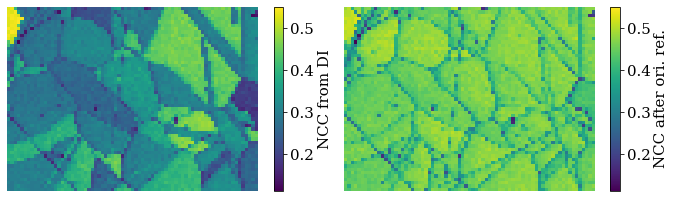Compare the histograms

In :
bins = np.linspace(vmin, vmax, 100)
fig, ax = plt.subplots(figsize=(9, 5))
_ = ax.hist(ncc_map.ravel(), bins, alpha=0.5, label="NCC from DI")
_ = ax.hist(
ncc_after_orientation_refinement.ravel(),
bins,
alpha=0.5,
label=ncc_after_label,
)
ax.set_xlabel("Normalized cross correlation (NCC) scores")
ax.set_ylabel("Frequency")
ax.legend()
fig.tight_layout();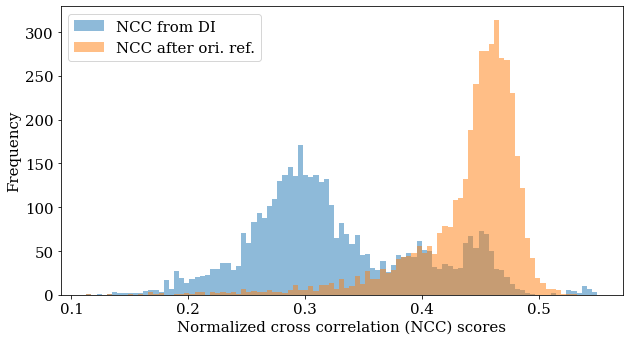We see that DI found the best orientation (with a fixed PC) for most of the patterns, which the refinement was able to improve further. However, there are a few patterns with a very low NCC score (0.1-0.2) which refinement couldn't improve upon, which tells us that these were misindexed during DI. If there are Kikuchi bands in these patterns, a larger dictionary with a finer orientation sampling could improve indexing of them.

Let's also plot the (IPF) $X$ orientation maps before and after refinement, and also the disorientation angle (smallest misorientation angle found after accounting for symmetry) difference between the two maps

In :
ori_ref = xmap_refined.orientations

ckey.direction = Vector3d.xvector()
rgb_z = ckey.orientation2color(ori)
rgb_z_ref = ckey.orientation2color(ori_ref)

fig, ax = plt.subplots(ncols=3, figsize=(14, 3))
ax.imshow(rgb_z.reshape(xmap.shape + (3,)))
ax.imshow(rgb_z_ref.reshape(xmap.shape + (3,)))
im2 = ax.imshow(mori_angle.reshape(xmap.shape))
fig.colorbar(im2, ax=ax, label=r"Disorientation angle $\omega$ [$^{\circ}$]")
for a in ax:
a.axis("off")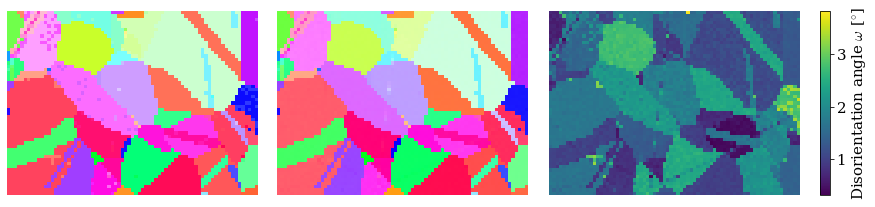We see that refinement was able to remove the scatter of similar colors in the grains.

### Refine projection centers¶

We use refine_projection_center() to refine PCs while keeping the orientations fixed, using the local modified Powell method. This method is also known as Bound Optimization BY Quadratic Approximation (BOBYQA), and is used in EMsoft and discussed by Singh et al. (2017). We will pass a trust_region of +/- 5% for the PC parameters to use as upper and lower bounds during refinement.

We can also pass compute=False, to do the refinement later. The results will then be a dask.array.Array. We can pass this array to kikuchipy.indexing.compute_refine_projection_center_results() and perform the refinement to retrieve the results

In :
result_array = s.refine_projection_center(
xmap=xmap,
detector=detector,
master_pattern=mp,
energy=energy,
method="minimize",
method_kwargs=dict(method="Powell", options=dict(ftol=1e-3)),
trust_region=[0.05, 0.05, 0.05],
compute=False,
)

Refinement information:
Local optimization method: Powell (minimize)
Keyword arguments passed to method: {'method': 'Powell', 'options': {'ftol': 0.001}}
Trust region: [0.05, 0.05, 0.05]

In :
ncc_after_pc_refinement, detector_refined = kp.indexing.compute_refine_projection_center_results(
results=result_array, detector=detector, xmap=xmap
)

Refining 4125 projection center(s):
[########################################] | 100% Completed |  1min 14.1s
Refinement speed: 55 patterns/s


Note that refine_orientation() and refine_orientation_projection_center() also takes the compute parameter, and there are similar functions to compute the refinement results: kikuchipy.indexing.compute_refine_orientation_results() and kikuchipy.indexing.compute_refine_orientation_projection_center_results().

Let's plot the refined scores and PCs

In :
ncc_pc_ref_min = np.min(ncc_after_pc_refinement)
ncc_pc_ref_max = np.max(ncc_after_pc_refinement)

vmin2 = min([ncc_di_min, ncc_pc_ref_min])
vmax2 = max([ncc_di_max, ncc_pc_ref_max])

In :
ncc_after_pc_label = "NCC after PC refinement"

fig, ax = plt.subplots(ncols=2, figsize=(10, 3))
im0 = ax.imshow(ncc_map, vmin=vmin2, vmax=vmax2)
im1 = ax.imshow(ncc_after_pc_refinement, vmin=vmin2, vmax=vmax2)
ax.axis("off")
ax.axis("off")
fig.colorbar(im0, ax=ax, label="NCC from DI")
fig.colorbar(im1, ax=ax, label=ncc_after_pc_label)
fig.tight_layout();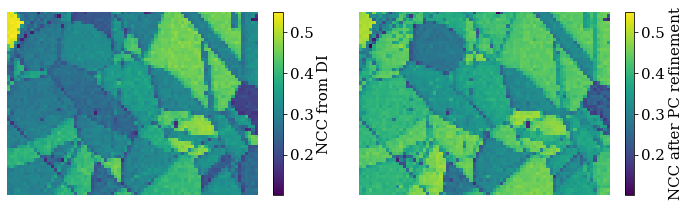Compare the histograms

In :
bins = np.linspace(vmin2, vmax2, 100)
fig, ax = plt.subplots(figsize=(9, 5))
_ = ax.hist(ncc_map.ravel(), bins, alpha=0.5, label="NCC from DI")
_ = ax.hist(
ncc_after_pc_refinement.ravel(),
bins,
alpha=0.5,
label=ncc_after_pc_label,
)
ax.set_xlabel("Normalized cross correlation (NCC) scores")
ax.set_ylabel("Frequency")
ax.legend()
fig.tight_layout();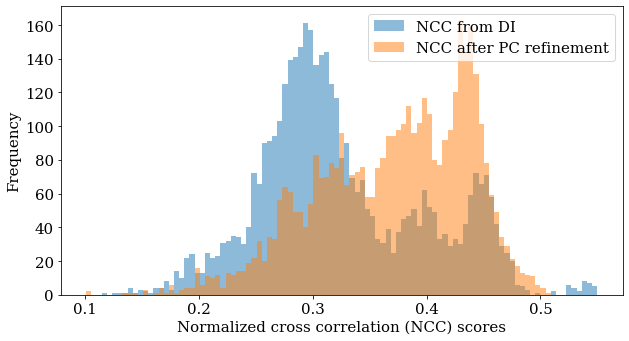In :
print(
f"PC used in DI:\t\t{detector.pc_average}\n"
f"PC after PC refinement:\t{detector_refined.pc_average}"
)

fig, ax = plt.subplots(ncols=3, figsize=(15, 3))
im0 = ax.imshow(detector_refined.pcx)
im1 = ax.imshow(detector_refined.pcy)
im2 = ax.imshow(detector_refined.pcz)
fig.colorbar(im1, ax=ax, label="Projection center y")
fig.colorbar(im0, ax=ax, label="Projection center x")
fig.colorbar(im2, ax=ax, label="Projection center z")
for a in ax:
a.axis("off")
fig.tight_layout();

PC used in DI:		[0.421 0.221 0.505]
PC after PC refinement:	[0.419 0.222 0.505]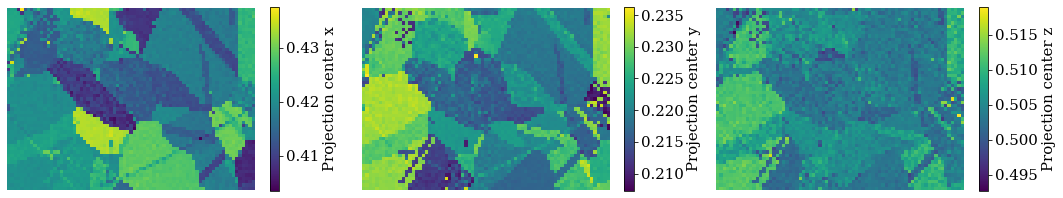### Refine orientations and projection centers¶

We use refine_orientation_projection_center() to refine orientations and PCs at the same time. For the purpose of saving time and computation resources in this demonstration, we'll do this for the upper left quarter of the data set only

In :
nav_shape = s.axes_manager.navigation_shape[::-1]
y1, x1 = np.array(nav_shape) // 2
slices2d = (slice(0, y1), slice(0, x1))

In :
s2 = s.inav[slices2d[::-1]]  # HyperSpy flips the usual NumPy (row, column) to (column, row)
s2

Out:
<EBSD, title: patterns Scan 1, dimensions: (37, 27|60, 60)>
In :
xmap2 = xmap[slices2d]
xmap2

Out:
Phase  Orientations  Name  Space group  Point group  Proper point group     Color
0  999 (100.0%)    ni        Fm-3m         m-3m                 432  tab:blue
Properties: scores, simulation_indices
Scan unit: um
In :
xmap_refined2, detector_refined2 = s2.refine_orientation_projection_center(
xmap=xmap2,
detector=detector,
master_pattern=mp,
energy=energy,
method="minimize",
method_kwargs=dict(options=dict(fatol=1e-3)),
compute=True,
)

Refinement information:
Keyword arguments passed to method: {'options': {'fatol': 0.001}, 'method': 'Nelder-Mead'}
Refining 999 orientation(s) and projection center(s):
[########################################] | 100% Completed |  1min 18.4s
Refinement speed: 12 patterns/s

In :
xmap_refined2

Out:
Phase  Orientations  Name  Space group  Point group  Proper point group     Color
0  999 (100.0%)    ni        Fm-3m         m-3m                 432  tab:blue
Properties: scores
Scan unit: um

Compare the NCC score maps. We use the same colorbar bounds for both maps

In :
ncc_after_orientation_pc_refinement = xmap_refined2.get_map_data("scores")
ncc_map2 = ncc_map[slices2d]

ncc2_di_min = np.min(ncc_map2)
ncc2_di_max = np.max(ncc_map2)
ncc_ori_pc_ref_min = np.min(ncc_after_orientation_pc_refinement)
ncc_ori_pc_ref_max = np.max(ncc_after_orientation_pc_refinement)

vmin3 = min([ncc2_di_min, ncc_ori_pc_ref_min])
vmax3 = max([ncc2_di_max, ncc_ori_pc_ref_max])

In :
ncc_after_ori_pc_label = "NCC after ori./PC ref."

fig, ax = plt.subplots(ncols=2, figsize=(9, 3))
im0 = ax.imshow(ncc_map2, vmin=vmin, vmax=vmax)
im1 = ax.imshow(ncc_after_orientation_pc_refinement, vmin=vmin3, vmax=vmax3)
fig.colorbar(im0, ax=ax, label="NCC from DI")
fig.colorbar(im1, ax=ax, label=ncc_after_ori_pc_label)
ax.axis("off")
ax.axis("off")
fig.tight_layout();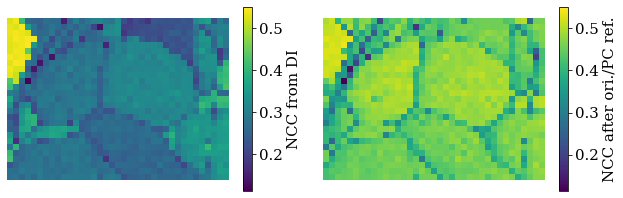Compare the histograms

In :
bins = np.linspace(vmin3, vmax3, 100)
fig, ax = plt.subplots(figsize=(9, 5))
_ = ax.hist(ncc_map2.ravel(), bins, alpha=0.5, label="NCC from DI")
_ = ax.hist(
ncc_after_orientation_pc_refinement.ravel(),
bins,
alpha=0.5,
label=ncc_after_ori_pc_label,
)
ax.set_xlabel("Normalized cross correlation (NCC) scores")
ax.set_ylabel("Frequency")
ax.legend()
fig.tight_layout();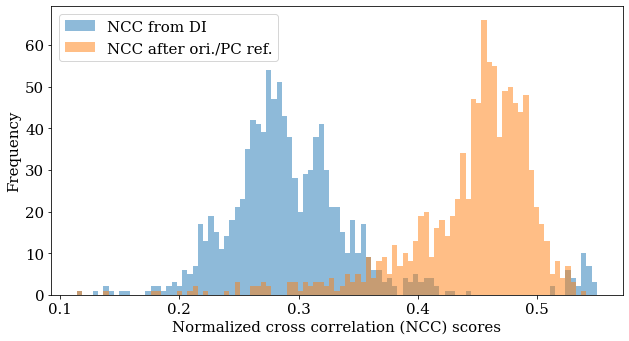Let's also inspect the refined PC parameters

In :
print(
f"PC used in DI:\t\t{detector.pc_average}\n"
f"PC after PC refinement:\t{detector_refined2.pc_average}"
)

pc_to_plot = (detector_refined2.pcx, detector_refined2.pcy, detector_refined2.pcz)
fig, ax = plt.subplots(ncols=3, figsize=(15, 3))
for a, to_plot, label in zip(ax, pc_to_plot, ("x", "y", "z")):
im = a.imshow(to_plot)
fig.colorbar(im, ax=a, label=f"Projection center {label}")
a.axis("off")
fig.tight_layout();

PC used in DI:		[0.421 0.221 0.505]
PC after PC refinement:	[0.423 0.214 0.5  ]